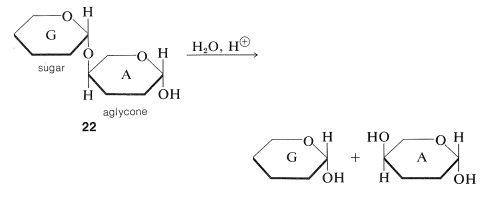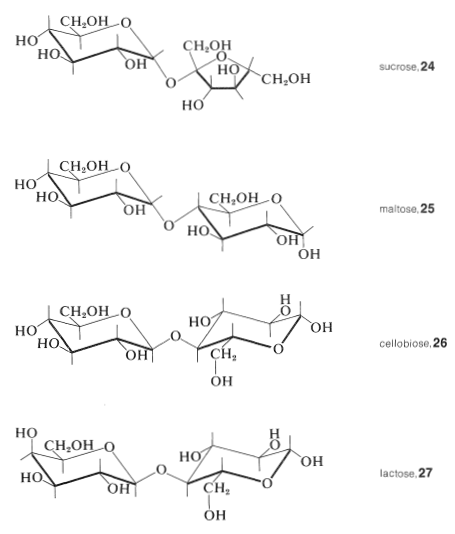# 20.7: Disaccharides

$$\newcommand{\vecs}{\overset { \rightharpoonup} {\mathbf{#1}} }$$ $$\newcommand{\vecd}{\overset{-\!-\!\rightharpoonup}{\vphantom{a}\smash {#1}}}$$$$\newcommand{\id}{\mathrm{id}}$$ $$\newcommand{\Span}{\mathrm{span}}$$ $$\newcommand{\kernel}{\mathrm{null}\,}$$ $$\newcommand{\range}{\mathrm{range}\,}$$ $$\newcommand{\RealPart}{\mathrm{Re}}$$ $$\newcommand{\ImaginaryPart}{\mathrm{Im}}$$ $$\newcommand{\Argument}{\mathrm{Arg}}$$ $$\newcommand{\norm}{\| #1 \|}$$ $$\newcommand{\inner}{\langle #1, #2 \rangle}$$ $$\newcommand{\Span}{\mathrm{span}}$$ $$\newcommand{\id}{\mathrm{id}}$$ $$\newcommand{\Span}{\mathrm{span}}$$ $$\newcommand{\kernel}{\mathrm{null}\,}$$ $$\newcommand{\range}{\mathrm{range}\,}$$ $$\newcommand{\RealPart}{\mathrm{Re}}$$ $$\newcommand{\ImaginaryPart}{\mathrm{Im}}$$ $$\newcommand{\Argument}{\mathrm{Arg}}$$ $$\newcommand{\norm}{\| #1 \|}$$ $$\newcommand{\inner}{\langle #1, #2 \rangle}$$ $$\newcommand{\Span}{\mathrm{span}}$$$$\newcommand{\AA}{\unicode[.8,0]{x212B}}$$

## General Types and Properties

Combinations of two or more of the simple sugars through glycoside linkages give substances known as polysaccharides. They also are called oligosaccharides if made from two to ten sugar units. The simplest oligosaccharides are disaccharides made of two molecules of simple sugars that can be joined with $$\ce{O}$$-glycoside links, and it probably is easiest to visualize these as shown in the "stripped-down" formulas, $$22$$ and $$23$$:You should look at $$22$$ and $$23$$ carefully to be sure that you recognize the difference between them.$$^5$$ In $$22$$, sugar A is acting as a simple hydroxy compound, the aglycone of the sugar G to which it is linked by an $$\ce{O}$$-glycoside linkage.$$^6$$ Hydrolysis of $$22$$ at the glycoside link then will proceed as follows:Disaccharides such as $$22$$ are like glucose in being reducing sugars (Section 20-2B), because Component A has the hemiacetal grouping that is opened easily to the aldehyde form in the mildly alkaline conditions used for the Tollen's and Fehling's solution oxidations. Because there is a free hemiacetal group, reducing sugars also form osazones and they mutarotate (Sections 20-4B and 20-2C).

Disaccharides of type $$23$$ are different in that each sugar, G and G', is acting as both a glycoside sugar and as an aglycone. The linkage between them is that of a double-barreled acetal, $$\ce{-O-C-O-C-O}-$$, and there is no hemiacetal grouping in the molecule. Therefore these are nonreducing sugars as far as the standard tests go. However, hydrolysis of the $$\ce{O}$$-glycoside linkages of $$23$$ does generate reducing sugars with hemiacetal carbons:In general, we find that the nonreducing disaccharides give none of the carbonyl reactions observed for glucose, such as mutarotation and osazone formation, except when the conditions are sufficiently acidic to hydrolyze the acetal linkage.

Among the more important disaccharides are sucrose, $$24$$, maltose, $$25$$, cellobiose, $$26$$, and lactose, $$27$$:Sucrose and lactose occur widely as the free sugars, lactose in the milk of mammals, and sucrose in fruit and plants (especially in sugar cane and sugar beet). Maltose is the product of enzymatic hydrolysis of starch, and cellobiose is a product of hydrolysis of cellulose.

To fully establish the structure of a disaccharide, we must determine (1) the identity of the component monosaccharides; (2) the type of ring junction, furanose or pyranose, in each monosaccharide, as it exists in the disaccharide; (3) the positions that link one monosaccharide with the other; and (4) the anomeric configuration ($$\alpha$$ or $$\beta$$) of this linkage.

Hydrolysis of disaccharides with enzymes is very helpful in establishing anomeric configurations, because enzymes are highly specific catalysts for hydrolysis of the different types of glycosidic linkages. For instance, $$\alpha$$-$$D$$-glucosidase (maltase) catalyzes hydrolysis of $$\alpha$$-$$D$$-glycosides more rapidly than of $$\beta$$-$$D$$-glycosides. The enzyme emulsin (found in bitter almonds) in contrast shows a strong preference for $$\beta$$-$$D$$-glycosides over $$\alpha$$-$$D$$-glycosides. Yeast invertase catalyzes hydrolysis of $$\beta$$-$$D$$-fructosides.

## Structure of Sucrose

We know that sucrose consists of the two monosaccharides glucose and fructose because hydrolysis with acids or enzymes gives equal amounts of each hexose. Further, sucrose is not a reducing sugar, it forms no phenylosazone derivative, and it does not mutarotate. Therefore the anomeric carbons of both glucose and fructose must be linked through an oxygen bridge in sucrose. Thus sucrose is a glycosyl fructoside or, equally, a fructosyl glucoside.

Because sucrose is hydrolyzed by enzymes that specifically assist hydrolysis of both $$\alpha$$ glycosides (such as yeast $$\alpha$$-glucosidase) and $$\beta$$-fructosides (such as invertase), it is inferred that the glucose residue is present as an $$\alpha$$ glucoside and the fructose residue as a $$\beta$$ fructoside. If so, the remaining uncertainty in the structure of sucrose is the size of the rings in the glucose and fructose residues.

The size of the sugar rings in sucrose has been determined by the reactions shown in Figure 20-5. Methylation of sucrose with dimethyl sulfate in basic solution followed by hydrolysis of the octamethyl derivative gives 2,3,4,6-tetra-$$\ce{O}$$-methyl-$$D$$-glucopyranose (Section 20-4) and a tetra-$$\ce{O}$$-methyl-$$D$$-fructose. This establishes the glucose reside in sucrose as a glucopyranose. The fructose residue must be a fructofuranose because periodate oxidation of sucrose consumes three moles of periodate, whereby one mole of methanoic acid and one mole of a tetraaldehyde are formed. On bromine oxidation followed by acid hydrolysis, the tetraaldehyde gives 3-hydroxy-2-oxopropanoic acid (hydroxypyruvic acid, $$\ce{HOCH_2COCO_2H}$$), oxoethanoic acid (glyoxylic acid, $$\ce{OCHCO_2H}$$), and $$D$$-glyceric acid $$\left( \ce{HOCH_2CHOHCO_2H} \right)$$. Sucrose therefore has structure $$24$$, and this structure was confirmed by synthesis (R. Lemieux in 1953).Figure 20-5: Summary of reactions used to establish the ring structure of sucrose.

$$^5$$For now, we will ignore the possibility of different anomers of the disaccharide or their component sugars.

$$^6$$The manner in which sugars are linked together to form oligosaccharides was elucidated by W. N. Haworth, who received the Nobel Prize in chemistry in 1937 for this and other contributions to research on the structures and reactions of carbohydrates.